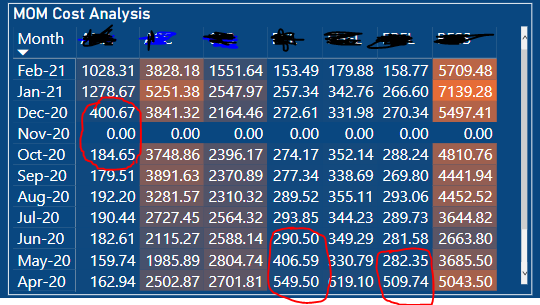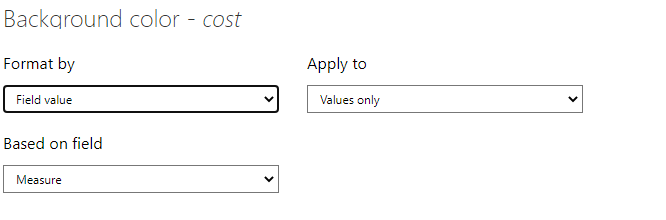cancel
Showing results for
Did you mean:Anonymous
Not applicable

## Power BI : Matrix Conditional Formatting for each column

Hi All,

I have a specific requirement where conditional formatting is required to highlight unusual cost/expenditure. I have used a matrix visual in it, and applied conditional formatting to it. But conditional formatting is applied across all the values. Please refer below snapshot of the visual, I have highlighted few cases in Red, which has a difference of 40-45% , but still same color is applied due to lot of other values.I need to apply conditional formatting for each column instead of across rows and columns.

1 ACCEPTED SOLUTIONCommunity Support

Hi, @Anonymous

The key is not in a certain column, each value in the matrix is originally related to the row and column, as long as the logic is correct. You can try to create a measure, then use it in conditional formatting.

Like this:

``````Measure =
VAR a =
SUMX (
FILTER (
ALL ( 'Table' ),
[account] = SELECTEDVALUE ( 'Table'[account] )
&& [month] = SELECTEDVALUE ( 'Table'[month] )
),
[cost]
)
RETURN
IF ( a < 1000, "Red" )``````Best Regards

Janey Guo

If this post helps, then please consider Accept it as the solution to help the other members find it more quickly.

5 REPLIES 5Community Support

Hi, @Anonymous

Has your problem solved? The conditional format can be customized, the key depends on your logic of ‘highlight unusual cost/expenditure’.

Can you share the logic you want and your desult result like?

Best Regards

Janey GuoAnonymous
Not applicable

@v-janeyg-msft  Let me explain my scenario in detail. Below is my sample raw data, which tells me month wise cost of each account.I have chosen matrix visual to represent above data with conditional formatting on cost.

Under 'Column' section of matrix visual, I have put Account column. Under 'Values' section I have put Cost. What problem I am facing is that, if you closely observe above data, Account 1 cost has varried from 100 to 400 which is significant but since rest of the Account costs is always above 1000, all Account 1 values are falling under same color code when put under conditional formatting.
I have highlighted such actual scenarios in my original post.

So I was wondering, whether we can have conditional formatting for each column rather than across rows and columns.Community Support

Hi, @Anonymous

The key is not in a certain column, each value in the matrix is originally related to the row and column, as long as the logic is correct. You can try to create a measure, then use it in conditional formatting.

Like this:

``````Measure =
VAR a =
SUMX (
FILTER (
ALL ( 'Table' ),
[account] = SELECTEDVALUE ( 'Table'[account] )
&& [month] = SELECTEDVALUE ( 'Table'[month] )
),
[cost]
)
RETURN
IF ( a < 1000, "Red" )``````Best Regards

Janey Guo

If this post helps, then please consider Accept it as the solution to help the other members find it more quickly.Frequent Visitor

Hi Janey,

Thanks for the solution. However, if I want to apply individual formatting to each column separately based on a range. How would I do that?
For Example, I want to highlight each column in a different shade of color based on a condition such as follows: If account1(value) >100 and <500 then Light Red else if account1(value) > 500 and <1000 then Purple else if account1(value) >1000 and <1500 then light green.Super User

@Anonymous , Are you column(pivot) ?

You can create a measure like these and use in conditional formatting with "Field Value" option

if(Max(Table[Col]) = "XYZ" , "red", "blue")

or more like example

Color = if(FIRSTNONBLANK('Table'[Year],2014) <=2016 && AVERAGE(Sales[Sales Amount])<170
,"lightgreen",if(FIRSTNONBLANK('Table'[Year],2014)>2018,"red","yellow"))
Color sales = if([Sales Today] -[sales yesterday]>0,"green","red")

color =
switch ( true(),
FIRSTNONBLANK('Table'[commodity],"NA") ="commodity1" && sum('Table'[Value]) >500,"lightgreen",
FIRSTNONBLANK('Table'[commodity],"NA") ="commodity2" && sum('Table'[Value]) >1000,"lightgreen",
"red"
)

steps

!! Microsoft Fabric !!
Microsoft Power BI Learning Resources, 2023 !!
Learn Power BI - Full Course with Dec-2022, with Window, Index, Offset, 100+ Topics !!
Did I answer your question? Mark my post as a solution! Appreciate your Kudos !! Proud to be a Super User! !!